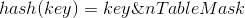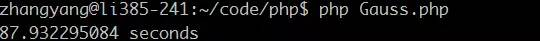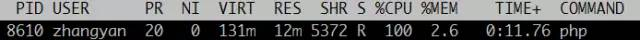# 略微加速

## 略速 - 互联网笔记

PHP哈希表碰撞攻击原理

2017-06-12 leiting (4336阅读)

PHP是使用单链表存储碰撞的数据，因此实际上PHP哈希表的平均查找复杂度为O(L)，其中L为桶链表的平均长度;而最坏复杂度为O(N)，此时所有数据全部碰撞，哈希表退化成单链表。下图PHP中正常哈希表和退化哈希表的示意图。Zend哈希表的内部实现

PHP中使用一个叫Backet的结构体表示桶，同一哈希值的所有桶被组织为一个单链表。哈希表使用HashTable结构体表示。相关源码在zend/Zend_hash.h下：

```typedef struct bucket {
ulong h;                        /* Used for numeric indexing */
uint nKeyLength;
void *pData;
void *pDataPtr;
struct bucket *pListNext;
struct bucket *pListLast;
struct bucket *pNext;
struct bucket *pLast;
char arKey; /* Must be last element */
} Bucket;

typedef struct _hashtable {
uint nTableSize;
uint nNumOfElements;
ulong nNextFreeElement;
Bucket *pInternalPointer;   /* Used for element traversal */
Bucket *pListTail;
Bucket **arBuckets;
dtor_func_t pDestructor;
zend_bool persistent;
unsigned char nApplyCount;
zend_bool bApplyProtection;
#if ZEND_DEBUG
int inconsistent;
#endif

} HashTable;```

```ZEND_API int _zend_hash_init(HashTable *ht, uint nSize, hash_func_t pHashFunction, dtor_func_t pDestructor, zend_bool persistent ZEND_FILE_LINE_DC)
{
uint i = 3;
Bucket **tmp;

SET_INCONSISTENT(HT_OK);

//长度向2的整数次幂圆整
if (nSize >= 0x80000000) {
/* prevent overflow */
ht->nTableSize = 0x80000000;
} else {
while ((1U << i) < nSize) {
i++;
}
ht->nTableSize = 1 << i;
}

ht->nTableMask = ht->nTableSize - 1;

/*此处省略若干代码…*/

return SUCCESS;

}```

Zend HashTable的哈希算法异常简单：```ZEND_API int zend_hash_index_find(const HashTable *ht, ulong h, void **pData)
{
uint nIndex;
Bucket *p;

IS_CONSISTENT(ht);

nIndex = h & ht->nTableMask;

p = ht->arBuckets[nIndex];
while (p != NULL) {
if ((p->h == h) && (p->nKeyLength == 0)) {
*pData = p->pData;
return SUCCESS;
}
p = p->pNext;
}
return FAILURE;
}

ZEND_API int zend_hash_find(const HashTable *ht, const char *arKey, uint nKeyLength, void **pData)
{
ulong h;
uint nIndex;
Bucket *p;

IS_CONSISTENT(ht);

h = zend_inline_hash_func(arKey, nKeyLength);
nIndex = h & ht->nTableMask;

p = ht->arBuckets[nIndex];
while (p != NULL) {
if ((p->h == h) && (p->nKeyLength == nKeyLength)) {
if (!memcmp(p->arKey, arKey, nKeyLength)) {
*pData = p->pData;
return SUCCESS;
}
}
p = p->pNext;
}
return FAILURE;

}```

0000 0000 0000 0000 0000 & 0 1111 1111 1111 1111 = 0

0001 0000 0000 0000 0000 & 0 1111 1111 1111 1111 = 0

0010 0000 0000 0000 0000 & 0 1111 1111 1111 1111 = 0

0011 0000 0000 0000 0000 & 0 1111 1111 1111 1111 = 0

0100 0000 0000 0000 0000 & 0 1111 1111 1111 1111 = 0

……

```<?php

\$size = pow(2, 16);

\$startTime = microtime(true);

\$array = array();
for (\$key = 0, \$maxKey = (\$size - 1) * \$size; \$key <= \$maxKey; \$key += \$size) {
\$array[\$key] = 0;
}

\$endTime = microtime(true);

echo \$endTime - \$startTime, ' seconds', "\n";``````<?php

\$size = pow(2, 16);

\$startTime = microtime(true);

\$array = array();
for (\$key = 0, \$maxKey = (\$size - 1) * \$size; \$key <= \$size; \$key += 1) {
\$array[\$key] = 0;
}

\$endTime = microtime(true);

echo \$endTime - \$startTime, ' seconds', "\n";```POST攻击

POST攻击的防护